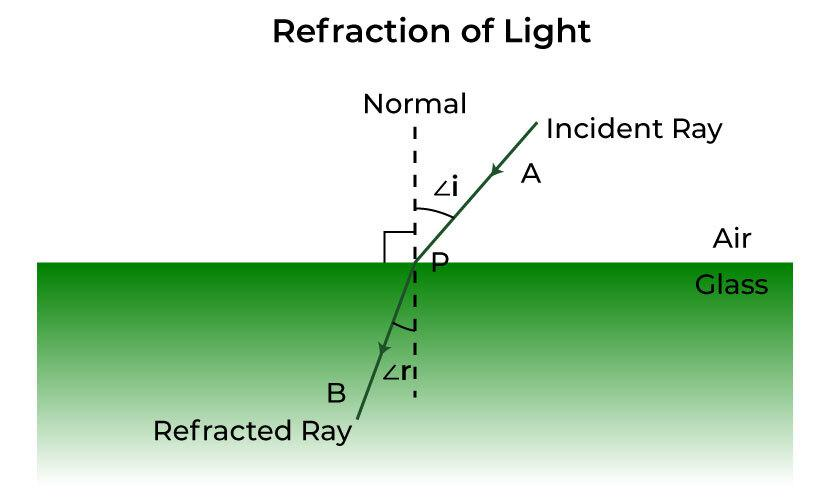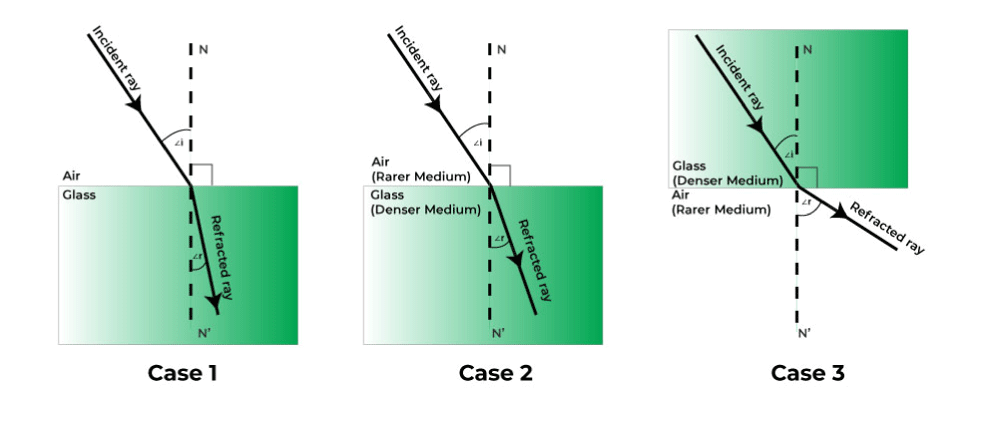Open in App
Not now

# Laws of Refraction of Light

• Difficulty Level : Easy
• Last Updated : 19 Dec, 2022

When you put a pencil in a glass of water, you might have seen that the pencil is slightly bent but actually it is not. You might have also observed that during summer, the trees and houses on the other side of an open ground appear to be shaking. While traveling on a road on a hot summer day, you might have seen that water appears in the middle of the road out of nowhere and when you get closer, there is no water. You might have also observed the apparent shift in the position of the sun at sunrise and sunset. The reason behind all this is the “Refraction of light“. We will discuss the Refraction in this article.

## What is Refraction of Light?

The phenomenon of change in the direction/path of light rays when it travels from one transparent medium to another is called refraction of light.

The bending of light is caused by the change in the wave’s speed. light has different speeds in different mediums. When light travels in the same medium, it travels along a straight line but when the medium has changed the speed of light changes and it bends.

## Terms Related to Refraction of Light• Incident ray: The ray of light falling to the surface at point P (above fig.) is called an incident ray (AP).
• Refracted ray: The ray of light traveling in glass with a change in direction is called refracted ray (PB).
• Angle of incidence (i): The angle formed between the incident ray and the normal is called the angle of incidence.
• Angle of refraction (r): The angle formed between normal and the angle of refraction is called the angle of refraction.

## Illustrations on Refraction of Light• Figure 1: Angle of incidence is greater than the angle of refraction (∠i > ∠r). When a ray of light travels from a rarer medium to a denser medium, it bends towards normal. Air rarer medium and glass is a denser medium.
• Figure 2: Angle of incidence is smaller than the angle of refraction (∠i <∠r). When a ray of light travels from a denser medium(glass) to a rarer medium(air), it bends away from normal.
• Figure 3: When a ray of light traveling along with normal shows no deflection. ∠i=∠r=0.

## Laws of Refraction of Light

### First Law of Refraction of Light

It states that the incident ray, refracted ray, and normal to the interface at the point of incidence all lie in the same plane.

### Second Law of Refraction of Light

It states that the ratio of the sine of the angle of incidence (i) and the sine of the angle of refraction (r) is constant. It is given as,

sin i / sin r = Constant

### Snell’s Law

Snell’s Law states that for the light if given colour and for the given pair of media, the ratio of the sine of the angle of incidence to the sine of the angle of refraction is constant. It gives the degree of refraction and also tells the relation between the angle of incidence, the angle of refraction and the refractive index of media.

sin i / sin r = μ = constant

where,

• μ is the refractive index of the medium.

## Refractive Index

Refractive index (for speed): The ratio of the speed of light in a vacuum to the speed of light in a given medium for the given wavelength is called the “Refractive index” of that medium. It is denoted by “μ“.

μ = c/v

where,

• c is the speed of light in a vacuum, and
• v is the speed of light in a medium.

Refractive index (for wavelength): The ratio of the wavelength of light in a vacuum to its wavelength in the given medium is called the “Refractive index” of that medium. It is denoted by “μ

μ = λvm

where.

• λv is the wavelength in a vacuum, and
• λm is the wavelength in a medium.

The Refractive index has no units. And the Refractive index of the medium depends upon:

• Wavelength of light
• Nature of the medium
• Nature of surrounding
• Temperature

## Applications of Refraction of Light

• Used for making peepholes in the doors, binoculars, cameras, lenses in projectors, etc.
• Used to correct the refractive errors of human eyes.
• Used for magnifying the image of an object or reducing the size of the image of an object.

## Solved Examples on Laws of Refraction of Light

Example 1: The refractive index of air to water is 5/3. The angle of incidence is sin 50 °. Find the angle of refraction.

Solution:

Given that,

i = 50°

μ = 5/3

Since,

μ = sin 50°/ sin r

sin r = sin 50° × (3/5)

= 0.766 × (3/5)

= 0.4596

r = sin-1(0.4596)

= 27.36°

Therefore, the angle of refraction will be 27.36°.

Example 2: The velocity of light in glass is 2 × 108m/s and the velocity of light in air is 3 × 108m/s. Find the refractive index of glass.

Solution:

Given that,

v = 2 × 108m/s

c = 3 × 108m/s

Since,

μ = c/v

Therefore,

μ = 3 × 108/ 2 ×108.

= 1.5

Therefore, refractive index will be 1.5

Example 3: Light traveling through an optical fiber (n=1.45) incident at the end of the fiber at 30°. Determine the angle of refraction beyond the fiber.

Solution:

Given that,

The Refractive Index of the Fiber, μ is 1.45

The angle of incidence, ∠i is 30°.

Since,

μ1 × sin i = μ2 × sin r

1.45 × sin 30° = 1 × sin r

1.45 × 1/2 = sin r

r = 46.46°

Question 4: Consider a ray is refracted at an angle of 20° and the refractive index is 1.5. Determine the angle of incidence by the ray.

Given that,

The Refractive Index, μ is 1.5

The angle of refraction, ∠r is 20°.

Since,

μ = sin i / sin r

1.5 = sin i / sin 20°

sin i = 0.513

i = 30.86°

## FAQs on Laws of Refraction of Light

Question 1: What is the difference between refraction and reflection of light?

Reflection: The bouncing back of light from a smooth and shining surface is called reflection. Angle of incidence is equal to the angle of reflection.  ∠i = ∠r.

Refraction: The bending of light rays, when it travels from one medium to another is called refraction of light. The angle of incidence and the refracted ray may or may not be equal.

Question 2: What do you mean by Refraction of Light?

The phenomenon of change in the direction/path of light rays when it travels from one transparent medium to another is called refraction of light.

Question 3: Why does the sun near the horizon appear flattened at sunrise and sunset?

The horizon appears flattened due to atmospheric refraction. The density and refractive index of the atmosphere decreases with increasing altitude. So, the rays of sun from the top portion and bottom portion are refracted by different degrees.

Question 4: Why do stars twinkle?

The stars twinkle due to the atmospheric refraction. The stars’ light under goes undergoes several refractions before reaching the earth, refraction regularly changing the refractive index that’s why it twinkles.

Question 5: Why does a ray of light bend towards normal as it passes from air to glass?